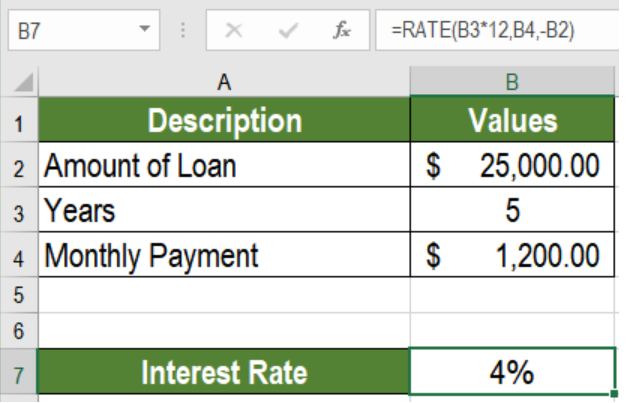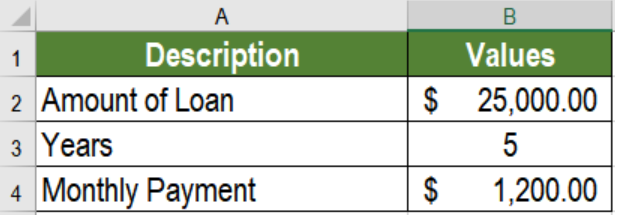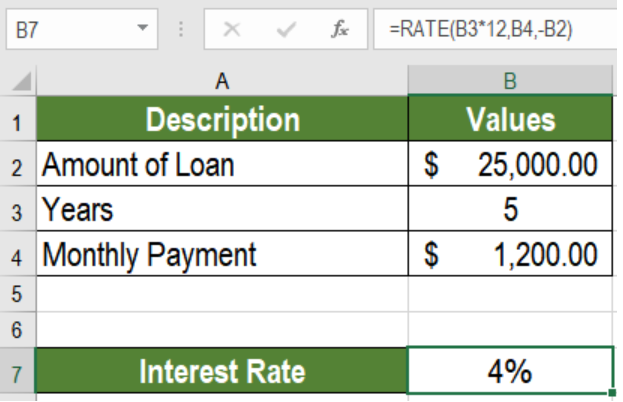Get instant live expert help with Excel or Google Sheets“My Excelchat expert helped me in less than 20 minutes, saving me what would have been 5 hours of work!”

#### Post your problem and you’ll get Expert help in seconds.

Your message must be at least 40 characters
Our professional Expert are available now. Your privacy is guaranteed.

# Excel RATE Function

Excel offers some great tools to work with interest. The Excel RATE function is one such tool. It is a financial function. It calculates the rate of interest for a given period of an investment or loan. In this tutorial, we will learn how to use the function RATE in Excel.Figure 1. Example of How to Use the Excel Rate Function

## Syntax & Arguments

`=RATE(nper, pmt, pv, [fv], [type], [guess])`

• nper – This argument is required. It is the count of period in total that we need to pay the amount.
• pmt – This argument is also required. It is the amount paid in each period. This value can not be changed during its course.
• pv – It is a required value as well. It represents the total amount of the investment or loan.
• fv – This value is optional. It represents the cash balance we want to achieve after last payment. Default is 0.
• type – This value is also optional. It indicates the time payments are due. 0 represents amounts are scheduled in the end of the period. 1 indicates amounts are due at the start. The default value is 0.
• guess – It is an optional value as well.  It is our guess at the rate. The default is 10%.

## Setting Up Data

The following example contains some sample values for a loan. Column A has the descriptions of the values. Column B has the years of the loan, the monthly payment and the amount of the loan.Figure 2. The Sample Data

To calculate the interest rate using the Excel RATE function in cell B5, we need to:

• Go to cell B7. Click on it with the mouse.
• Assign the formula `=RATE(B3*12,B4,-B2)` to B7.
• Press Enter.Figure 3. Applying the Formula

This will show the interest rate in cell B7 which is 4%.

## Notes

1. Excel calculates RATE by iterations. If the results of RATE do not converge to 0.0000001 after 20 iterations, it returns a #NUM! error. To prevent this, we may provide an initial ‘guess’ argument to the function.
2. Excel returns a #VALUE error if any of the arguments provided for RATE are non-numeric.
3. We need to change the number of periods or interest rate to months or quarters. We need to do this before calculating quarterly or monthly payments. Hence, we need to make sure nper has the correct units, i.e., quarters = 4*years, months = 12*years.

Most of the time, the problem you will need to solve will be more complex than a simple application of a formula or function. If you want to save hours of research and frustration, try our live Excelchat service! Our Excel Experts are available 24/7 to answer any Excel question you may have. We guarantee a connection within 30 seconds and a customized solution within 20 minutes.

### Did this post not answer your question? Get a solution from connecting with the expert.Another blog reader asked this question today on Excelchat:
Solution examplesI need a formula that has these 2 rules. If letter was rec'd between 1st and 15th = 1st of the month following letter rec'd date. If letter was rec'd between 16thst and end of the month= 1st of the 2nd month following letter rec'd date.
Solved by V. F. in 20 minsI need a column to total (Hours)(85) + (Travel)(.55) per line
Solved by F. Q. in 20 minsTrying to figure out what is wrong with my formula in Oct-ZD tab in column AY and BG.
Solved by O. Q. in 8 minsSo I have used vlookup to find the price of a product on a different sheet and then I have tried to times that by a quantity. e.g. =vlookup(d4,table35,2,false)*e4 This is the formula I typed in which worked for another list I did but for some reason it's not working now even though everything is practically the same, apart from the contents. Could you help?
Solved by B. U. in 60 minsI have a column containing date and time data together and i need to isolate the time data. When I tried to use text to column, it changed all the times to AM. There is an extremely large amount of data - over 550,000 rows so manually changing it won't work. The file is too large to attach.
Solved by S. E. in 40 mins## Subscribe to Excelchat.coAnother blog reader asked this question today on Excelchat: